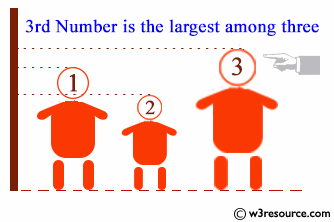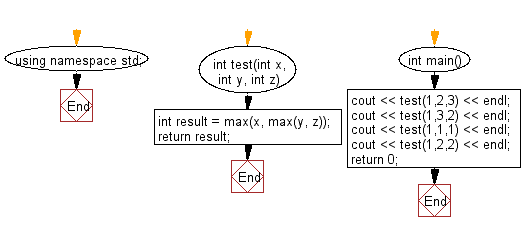﻿ C++ : Check the largest number among three given integers# C++ Exercises: Check the largest number among three given integers

## C++ Basic Algorithm: Exercise-18 with Solution

Write a C++ program to check the largest number among three given integers.

Sample Solution:

C++ Code :

``````#include <iostream>
using namespace std;

int test(int x, int y, int z)
{
int result = max(x, max(y, z));
return result;
}

int main()
{
cout << test(1,2,3) << endl;
cout << test(1,3,2) << endl;
cout << test(1,1,1) << endl;
cout << test(1,2,2) << endl;
return 0;
}
``````

Sample Output:

```3
3
1
2
```

Pictorial Presentation:Flowchart:C++ Code Editor:

Contribute your code and comments through Disqus.

What is the difficulty level of this exercise?

﻿